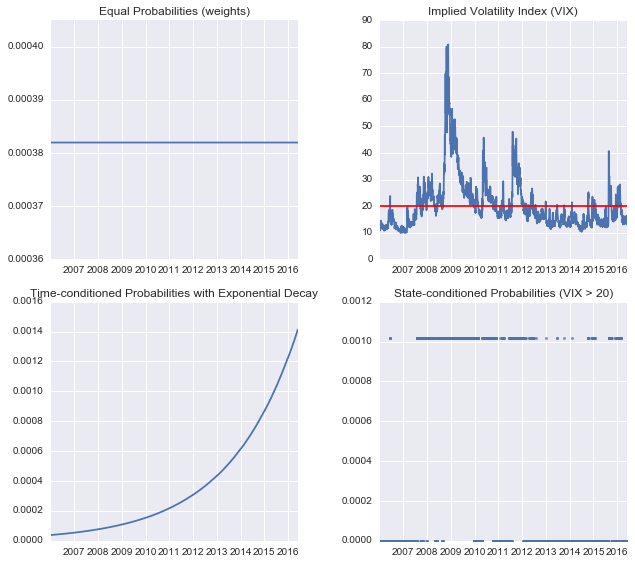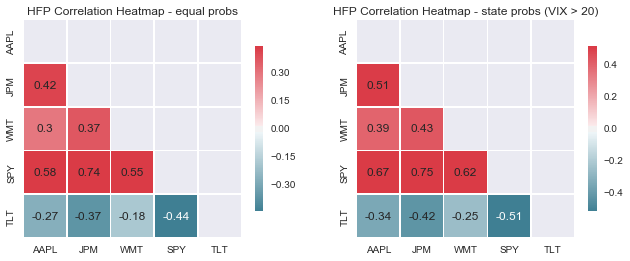# mini-Meucci : Applying The Checklist - Step 2

"Guessing before proving! Need I remind you that it is so that all important discoveries have been made?”
Henri Poincaré, French mathematician (1854-1912)

In this second leg of The Checklist tour, Estimation, we are going to make some educated guesses about the true unknown distribution of the invariants. But first...

## Recap and a bit more on Quest for Invariance

"The quest for invariance is necessary for the practitioners to learn about the future by observing the past in a stochastic environment."
The Prayer (former Checklist)

The essence is that the pattern (distribution) of the invariants will repeat.

"Being able to identify the invariants that steer the dynamics of the risk drivers is of crucial importance because it allows us to project the market randomness to the desired investment horizon."
The Prayer (former Checklist)

Also, recall that we chose a simple random walk to model the invariants (same model for all the stocks and ETFs). But if it isn't sufficient and your time series shows for example mean reversion or volatility clustering, then you should use more sophisticated models like ARMA or GARCH (see reference #3 at end of post).

## Estimation

Estimation is a yuge! topic - see chapter 4 of A. Meucci, 2005, Risk and Asset Allocation, (Springer) for an introduction.

In a nutshell, the goal is to find numbers (estimators) that describe the distribution of the invariants e.g. location and dispersion can be estimated by the sample mean and sample covariance matrix.

In general there are 3 approaches to Estimation: historical (non-parametric), analytical (parametric) and copula-marginal (mixed).

## Historical Approach

If you have enough data relative to your investment horizon (e.g. 10 yrs of daily data and a 21-day horizon), then it's recommended to go the historical non-parametric way, and this is the road we'll take throughout this tour - but with a twist!

"The simplest of all estimators for the invariants distribution is the nonparametric empirical distribution, justified by the law of large numbers, i.e. "i.i.d. history repeats itself". The empirical distribution assigns an equal probability to each of the past observations in the series of the historical scenarios."
The Prayer (former Checklist)

For an example of the analytical approach, see The Checklist slides (slide #7) where the 2-stock example assumes a bivariate normal distribution. For more on copula-marginal, go to the ARPM Bootcamp!

## Flexible Probabilities - the twist explained...

I put Flexible Probabilities into the must-see category of attractions in Meucci-land. Using them is a quick and powerful method of enhancing estimation techniques.

The twist is that instead of applying equal weight to all the historical observations/scenarios (as is common practice in calculating the sample mean and covariance), you can apply different weighting schemes. But make sure the weights are normalised to be like probabilities (i.e. range between 0 and 1 and sum to 1). In other words, use weighted estimators e.g. weighted mean and weighted covariance.

## Python Code Examples of Flexible Probabilities

The Python code below shows 2 examples of flexible probabilities:

1. Time-conditioned - apply higher weight to more recent observations, with the weights decaying exponentially
2. State-conditioned - using the VIX as an example, give weight to past scenarios where the VIX is greater than 20 and no weight otherwise
In :
%matplotlib inline
import numpy as np
import datetime
import math
import matplotlib.pyplot as plt
import matplotlib.gridspec as gridspec
import seaborn

# Get Yahoo data on 30 DJIA stocks and a few ETFs
tickers = ['MMM','AXP','AAPL','BA','CAT','CVX','CSCO','KO','DD','XOM','GE','GS',
'HD','INTC','IBM','JNJ','JPM','MCD','MRK','MSFT','NKE','PFE','PG',
'TRV','UNH','UTX','VZ','V','WMT','DIS','SPY','DIA','TLT','SHY']
start = datetime.datetime(2005, 12, 31)
end = datetime.datetime(2016, 5, 30)
rawdata = data.DataReader(tickers, 'yahoo', start, end)
risk_drivers = np.log(prices)
invariants = risk_drivers.diff().drop(risk_drivers.index)
T = len(invariants)

# Get VIX data
vix = data.DataReader('^VIX', 'yahoo', start, end)['Close']
vix.drop(vix.index, inplace=True)

# Equal probs
equal_probs = np.ones(len(vix)) / len(vix)

# Time-conditioned flexible probs with exponential decay
half_life = 252 * 2 # half life of 2 years
es_lambda = math.log(2) / half_life
exp_probs = np.exp(-es_lambda * (np.arange(0, len(vix))[::-1]))
exp_probs = exp_probs / sum(exp_probs)
# effective number of scenarios
ens_exp_probs = math.exp(sum(-exp_probs * np.log(exp_probs)))

# State-conditioned flexible probs based on VIX > 20
state_probs = np.zeros(len(vix)) / len(vix)
state_cond = np.array(vix > 20)
state_probs[state_cond] = 1 / state_cond.sum()

# Plot charts
fig = plt.figure(figsize=(9, 8))
gs = gridspec.GridSpec(2, 2)
ax.plot(vix.index, equal_probs)
ax.set_title("Equal Probabilities (weights)")
ax2.plot(vix.index, vix)
ax2.set_title("Implied Volatility Index (VIX)")
ax2.axhline(20, color='r')
ax3.plot(vix.index, exp_probs)
ax3.set_title("Time-conditioned Probabilities with Exponential Decay")
ax4.plot(vix.index, state_probs, marker='o', markersize=3, linestyle='None', alpha=0.7)
ax4.set_title("State-conditioned Probabilities (VIX > 20)")
plt.tight_layout()
plt.show()There are many other ways to create sets of flexible probabilities (e.g. rolling window), and indeed these can also be combined together using Meucci's Entropy-based technique (see reference #4 at end of post).

## Example Application of Historical with Flexible Probabilities Approach

Let's now apply the Historical with Flexible Probabilities (HFP) approach to perform a simple 'stress' analysis, and look at the difference between correlations using equal probabilities and state-conditioned probabilities when the VIX > 20 (stressed market condition)

To keep it simple, we'll choose a few tickers only.

In :
# Stress analysis
import rnr_meucci_functions as rnr

tmp_tickers = ['AAPL', 'JPM', 'WMT', 'SPY', 'TLT']

# HFP distribution of invariants using equal probs
mu, sigma2 = rnr.fp_mean_cov(invariants.ix[:,tmp_tickers].T, equal_probs)

# HFP distribution of invariants using state-conditioned probs (VIX > 20)
mu_s, sigma2_s = rnr.fp_mean_cov(invariants.ix[:,tmp_tickers].T, state_probs)

# Calculate correlations
from statsmodels.stats.moment_helpers import cov2corr
corr = cov2corr(sigma2)
corr_s = cov2corr(sigma2_s)

# Plot correlation heatmaps
rnr.plot_2_corr_heatmaps(corr, corr_s, tmp_tickers,
"HFP Correlation Heatmap - equal probs",
"HFP Correlation Heatmap - state probs (VIX > 20)")The main result is that when volatility is elevated (i.e. stressed market condition), correlations within equities rise, but they fall between bonds (TLT) and equities. This quantifies what we expect to see happen, and such scenario driven simulations are easily accomplished with the HFP approach. Of course, there are many more applications of HFP, and in the Aggregation step we'll look at a portfolio risk example.

Next stop on the tour is Projection...

1. The Checklist slides
2. The Prayer (former Checklist)
3. Review of Discrete and Continuous Processes in Finance: Theory and Applications http://symmys.com/node/131
4. Historical Scenarios with Fully Flexible Probabilities http://symmys.com/node/150